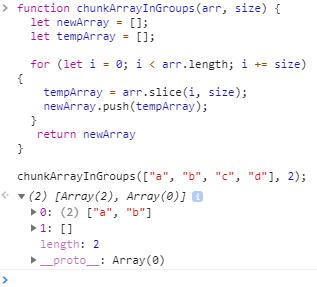# Code explanation in foor loop needed

Code explanation in foor loop needed
0

I just completed this challenge:
https://learn.freecodecamp.org/javascript-algorithms-and-data-structures/basic-algorithm-scripting/chunky-monkey/

In this challenge the objective is to “Write a function that splits an array (first argument) into groups the length of `size` (second argument) and returns them as a two-dimensional array.”

Here was my initial idea for the code to solve the problem:

``````function chunkArrayInGroups(arr, size) {
let newArray = [];
let tempArray = [];

for (let i = 0; i < arr.length; i += size) {
tempArray = arr.slice(i, size);
newArray.push(tempArray);
}
return newArray
}

chunkArrayInGroups(["a", "b", "c", "d"], 2);
``````

However I could only ever get it to push the first two elements (“a”, “b”) in the arr to newArray. A second array would seemingly be made, but it would be left empty.I then cheated a bit and looked at the solution in the hints section, and I saw that I only really needed to change this line of code:
`tempArray = arr.slice(i, size);`

to this:
`tempArray = arr.slice(i, i + size);`

It works now, but I don’t understand why. And I don’t understand why my initial code was insufficient. Initially i + size should be equal to 2 (i = 0 + 2). But on the second iteration of the loop i should be equal to 2 and i + size would therefore be 2 + 2 which is 4, which, if the array was longer would end up slicing too many elements from it, right?

Anyway, I’m confused. Please helpHello.

I’ll try to give you the most simple explanation that is, in your first solution without summing the i variable to the size, the size will always be 2 (in this case), since you’re using the variable passed, so you basically have to make the size follow along with the i variable.

Imagine this case

`chunkArrayInGroups(["a", "b", "c", "d","e","f","g","h"], 2);`

With your solution, the cycle would be

``````slice(0,2)
slice(2,2)
slice(4,2)
slice(6,2)
``````

You pass to the slice method where you want it to begin and where you want it to end, so you always have to add to the size the i , so with the correct solution it would be

``````slice(0,2)
slice(2,4)
slice(4,6)
slice(6,8)
``````

Now, this is right!
I hope this wasn’t too confusing!

1 Like

Of course! Thank you! I was thinking that the second parameter in slice was the number of elements to be copied (like when using splice where the second parameter is how many elements to delete from an array), but for slice it’s the index at which to stop the extraction.

1 Like